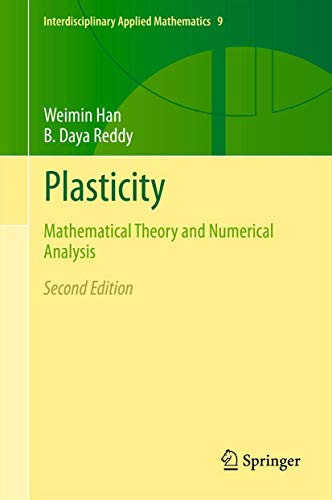• Free Shipping on all orders in Australia
• Over 7 million books in stock
• Proud to be B-Corp
• We aim to be carbon neutral by 2022
• Over 120,000 Trustpilot reviews
Item 1 of 0# Plasticity by Weimin Han

Condition - New
\$355.49
Only 2 left

## Summary

In its revised and expanded second edition, this book examines the theoretical aspects of small strain theory of elastoplasticity with hardening assumptions, offering a comprehensive and unified treatment of mathematical theory and numerical analysis.

This book will be specially printed for your order, which means it may take a little longer to arrive (5 days). We do this to cut down on waste & to help protect our planet.

## Plasticity Summary

### Plasticity: Mathematical Theory and Numerical Analysis by Weimin Han

This book focuses on the theoretical aspects of small strain theory of elastoplasticity with hardening assumptions. It provides a comprehensive and unified treatment of the mathematical theory and numerical analysis. It is divided into three parts, with the first part providing a detailed introduction to plasticity, the second part covering the mathematical analysis of the elasticity problem, and the third part devoted to error analysis of various semi-discrete and fully discrete approximations for variational formulations of the elastoplasticity. This revised and expanded edition includes material on single-crystal and strain-gradient plasticity. In addition, the entire book has been revised to make it more accessible to readers who are actively involved in computations but less so in numerical analysis. Reviews of earlier edition: "The authors have written an excellent book which can be recommended for specialists in plasticity who wish to know more about the mathematical theory, as well as those with a background in the mathematical sciences who seek a self-contained account of the mechanics and mathematics of plasticity theory." (ZAMM, 2002) "In summary, the book represents an impressive comprehensive overview of the mathematical approach to the theory and numerics of plasticity. Scientists as well as lecturers and graduate students will find the book very useful as a reference for research or for preparing courses in this field." (Technische Mechanik) "The book is professionally written and will be a useful reference to researchers and students interested in mathematical and numerical problems of plasticity. It represents a major contribution in the area of continuum mechanics and numerical analysis." (Math Reviews)

## Plasticity Reviews

"The book is unique in its broad consideration of analytical and numerical aspects of plasticity. ... The book is well written and carefully presented overall. It presents a wealth of useful material otherwise absent from other plasticity books. It exposes both the interested mechanician and the interested mathematician to mathematical problems in plasticity in a unified manner, albeit to be pursued in their own way." (Anurag Gupta, SIAM Review, Vol. 58 (4), December, 2016)

Preface to the Second Edition.- Preface to the First Edition.-Preliminaries.- Continuum Mechanics and Linearized Elasticity.- Elastoplastic Media.- The Plastic Flow Law in a Convex-Analytic Setting.- Basics of Functional Analysis and Function Spaces.- Variational Equations and Inequalities.- The Primal Variational Problem of Elastoplasticity.- The Dual Variational Problem of Classical Elastoplasticity.- Introduction to Finite Element Analysis.- Approximation of Variational Problems.- Approximations of the Abstract Problem.- Numerical Analysis of the Primal Problem.- References.- Index.-

NLS9781489995940
9781489995940
1489995943
Plasticity: Mathematical Theory and Numerical Analysis by Weimin Han
New
Paperback
Springer-Verlag New York Inc.
2014-12-13
424
N/A
Book picture is for illustrative purposes only, actual binding, cover or edition may vary.
This is a new book - be the first to read this copy. With untouched pages and a perfect binding, your brand new copy is ready to be opened for the first time